## Designing and Analyzing Linear Systems¶

Supplemental Julia Notebook

Prof. Doug James

## Example: Tikhonov regularization¶

In :
A = [1 1;
1 1.00001]   ## An ill-conditioned matrix
cond(A)

Out:
400002.0000028382
In :
b = [1; 0.99];

In :
x = A \ b

Out:
2-element Array{Float64,1}:
1001.0
-1000.0
In :
norm(A*x-b)/norm(b)

Out:
6.46964603432798e-15
In :
norm(x)

Out:
1414.920845833358

## Regularized solve¶

In :
α = 0.00000000001
B = A'*A + α*eye(2)  ## Regularized

Out:
2×2 Array{Float64,2}:
2.0      2.00001
2.00001  2.00002
In :
x = B \ (A'*b)  ## Solve Normal equations

Out:
2-element Array{Float64,1}:
1000.99
-999.995
In :
relErr = norm(A*x-b)/norm(b)

Out:
2.552917567500873e-8
In :
norm(x)

Out:
1414.9136610830515

## Cholesky Factorization¶

In :
?(chol)

search: chol cholfact cholfact! searchsortedlast CachingPool chop chown chomp


Out:
chol(A) -> U

Compute the Cholesky factorization of a positive definite matrix A and return the UpperTriangular matrix U such that A = U'U.

chol(x::Number) -> y

Compute the square root of a non-negative number x.

In :
chol(rand(3,3))

ArgumentError: matrix is not symmetric/Hermitian. This error can be avoided by calling chol(Hermitian(A)) which will ignore either the upper or lower triangle of the matrix.

in chol(::Array{Float64,2}) at ./linalg/cholesky.jl:166
In :
U = chol(eye(3))

Out:
3×3 UpperTriangular{Float64,Array{Float64,2}}:
1.0  0.0  0.0
⋅   1.0  0.0
⋅    ⋅   1.0
In :
U'*U

Out:
3×3 Array{Float64,2}:
1.0  0.0  0.0
0.0  1.0  0.0
0.0  0.0  1.0
In :
R = rand(3,3);
A = R' * R

Out:
3×3 Array{Float64,2}:
1.03716   0.485069  0.700163
0.485069  0.273739  0.295844
0.700163  0.295844  0.765217
In :
L = chol(A)'

Out:
3×3 LowerTriangular{Float64,Array{Float64,2}}:
1.01841     ⋅         ⋅
0.476301   0.216509   ⋅
0.687507  -0.14603   0.520793
In :
b=ones(3);

In :
A \ b

Out:
3-element Array{Float64,1}:
-6.84456
13.0564
2.5217 
In :
y = L \ b

Out:
3-element Array{Float64,1}:
0.981924
2.45859
1.31328 
In :
L' \ y

Out:
3-element Array{Float64,1}:
-6.84456
13.0564
2.5217 

## Cholesky on Sparse Matrices¶

In :
using PyPlot
N = 15;
R = sprand(N, N, 0.1);
A = R*R' + 1.e-7 * speye(N)

Out:
15×15 sparse matrix with 29 Float64 nonzero entries:
[1 ,  1]  =  1.0e-7
[2 ,  2]  =  0.959033
[4 ,  2]  =  0.0536958
[5 ,  2]  =  0.568696
[9 ,  2]  =  0.188129
[3 ,  3]  =  1.11993
[5 ,  3]  =  0.1869
[2 ,  4]  =  0.0536958
[4 ,  4]  =  0.131619
[9 ,  4]  =  0.237006
⋮
[4 ,  9]  =  0.237006
[9 ,  9]  =  2.02699
[10, 10]  =  0.807924
[12, 10]  =  0.349218
[11, 11]  =  1.0e-7
[10, 12]  =  0.349218
[12, 12]  =  0.150947
[6 , 13]  =  0.211823
[13, 13]  =  0.212114
[14, 14]  =  1.0e-7
[15, 15]  =  1.0e-7
In :
spy(A)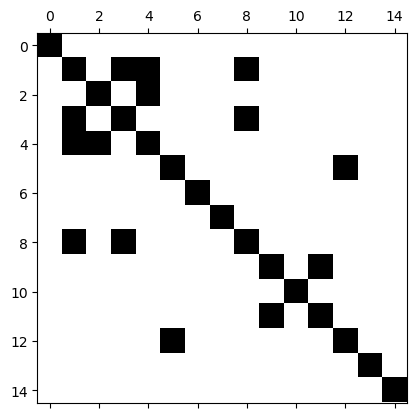Out:
PyObject <matplotlib.image.AxesImage object at 0x7fb20c898d50>
In :
svdvals(full(A))'

Out:
1×15 Array{Float64,2}:
2.09612  1.34565  1.09208  0.958871  …  1.0e-7  1.0e-7  1.0e-7  1.0e-7
In :
chol(A)

Use cholfact() instead of chol() for sparse matrices.

in chol(::SparseMatrixCSC{Float64,Int64}) at ./sparse/linalg.jl:918
In :
F = cholfact(A)

Out:
Base.SparseArrays.CHOLMOD.Factor{Float64}
type:          LLt
method: simplicial
maxnnz:         22
nnz:            22

In :
b = ones(N);
xF = F \ b

Out:
15-element Array{Float64,1}:
1.0e7
-2.92591
-0.0943949
9.39129
5.91608
6867.08
2.21476
1.0e7
-0.333173
-2.06776e6
1.0e7
4.78381e6
-6852.95
1.0e7
1.0e7    
In :
xA = A \ b   ## "\" is smart, and actually uses sparse Cholesky to solve system(!)

Out:
15-element Array{Float64,1}:
1.0e7
-2.92591
-0.0943949
9.39129
5.91608
6867.08
2.21476
1.0e7
-0.333173
-2.06776e6
1.0e7
4.78381e6
-6852.95
1.0e7
1.0e7    
In :
norm(xA-xF)/norm(xA)

Out:
2.5888015819409084e-11
In [ ]:



## 1D Laplacian matrix¶

In :
N=100;
A = spzeros(N,N)  ## sparse zero matrix

Out:
100×100 sparse matrix with 0 Float64 nonzero entries
In :
## Create 1D Laplacian matrix (h=1)
for i=1:N
A[i,i] = -2.;
if (i>1) A[i,i-1] = 1; end
if (i<N) A[i,i+1] = 1; end
end
A

Out:
100×100 sparse matrix with 298 Float64 nonzero entries:
[1  ,   1]  =  -2.0
[2  ,   1]  =  1.0
[1  ,   2]  =  1.0
[2  ,   2]  =  -2.0
[3  ,   2]  =  1.0
[2  ,   3]  =  1.0
[3  ,   3]  =  -2.0
[4  ,   3]  =  1.0
[3  ,   4]  =  1.0
[4  ,   4]  =  -2.0
⋮
[96 ,  97]  =  1.0
[97 ,  97]  =  -2.0
[98 ,  97]  =  1.0
[97 ,  98]  =  1.0
[98 ,  98]  =  -2.0
[99 ,  98]  =  1.0
[98 ,  99]  =  1.0
[99 ,  99]  =  -2.0
[100,  99]  =  1.0
[99 , 100]  =  1.0
[100, 100]  =  -2.0
In :
nnz(A)

Out:
298
In :
nnz(A)/(N*N)

Out:
0.0298
In [ ]:


In :
spy(A)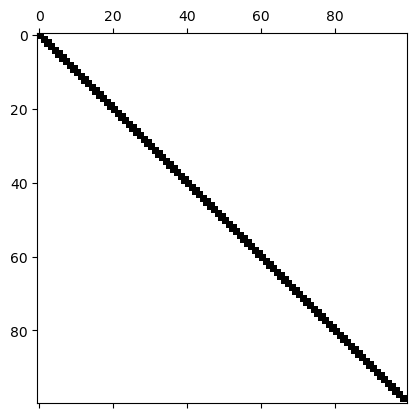Out:
PyObject <matplotlib.image.AxesImage object at 0x7fb20e6e0990>
In :
# SOLVE Laplaces equation for point charge:
#     A * potential = chargeDensity
chargeDensity = zeros(N); chargeDensity[div(N,2)] = 1.;  # point charge at N/2 position
plot(chargeDensity)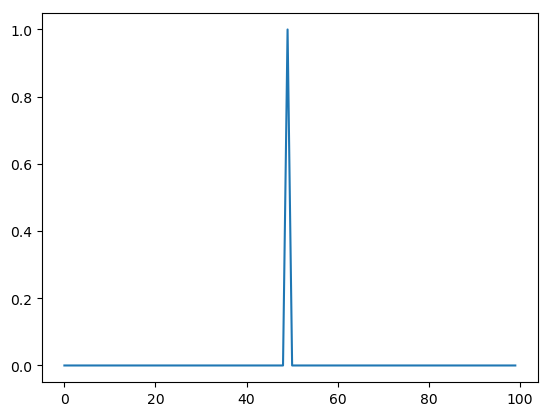Out:
1-element Array{Any,1}:
PyObject <matplotlib.lines.Line2D object at 0x7fb20e610310>
In :
plot(-A \ chargeDensity) # electric potential = solution to 1D Laplace's equation (-gradient --> electric field)
# Matrix A is actually only positive semidefinite (!)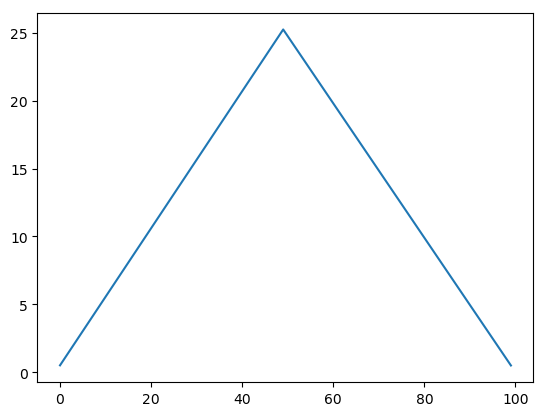Out:
1-element Array{Any,1}:
PyObject <matplotlib.lines.Line2D object at 0x7fb20e4851d0>

## Visualizing "Fill In"¶

In :
N = 15;
L = speye(N, N)
L[1:N,1] = ones(N);

In :
spy(L)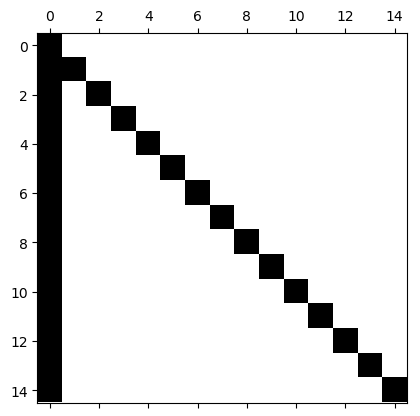Out:
PyObject <matplotlib.image.AxesImage object at 0x7fb20db9ae90>
In :
nnz(L)

Out:
29
In :
nnz(L*L')

Out:
225
In :
N*N

Out:
225
In :
## Random sparse lower triangular matrix
N = 225
L = tril(sprand(N,N,0.1) + speye(N))
print("nnz(L)/(N*N) = ",(nnz(L)/(N*N)))
spy(L)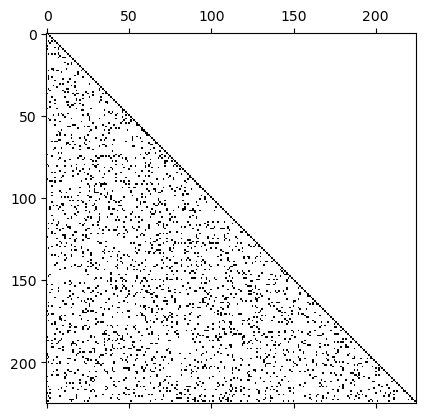nnz(L)/(N*N) = 0.052997530864197534
Out:
PyObject <matplotlib.image.AxesImage object at 0x7fb20ca34b10>
In :
print("nnz(L*L')/(N*N) = ",(nnz(L*L')/(N*N)))
spy(L*L')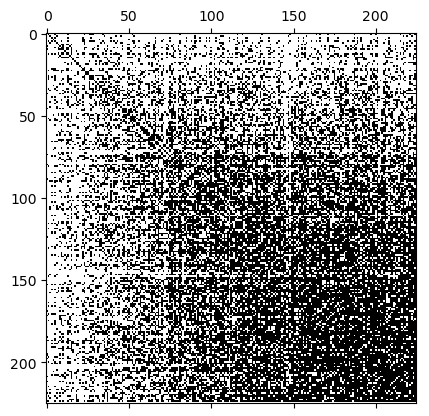nnz(L*L')/(N*N) = 0.5019456790123457
Out:
PyObject <matplotlib.image.AxesImage object at 0x7fb20c966810>
In [ ]: